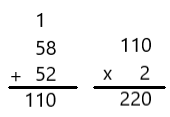# McGraw Hill Math Grade 4 Chapter 13 Lesson 6 Answer Key Problem Solving: Choosing a Method

Practice the questions of McGraw Hill Math Grade 4 Answer Key PDF Chapter 13 Lesson 6 Problem Solving: Choosing a Method to secure good marks & knowledge in the exams.

## McGraw-Hill Math Grade 4 Answer Key Chapter 13 Lesson 6 Problem Solving: Choosing a Method

Solve.

Tell which method you chose for each problem. Explain why.

Question 1.
Kameko and Bill have the same size water bottle. Kameko’s bottle is $$\frac{3}{10}$$ full of water. Bill’s bottle is $$\frac{5}{10}$$ full of water. How much more water does Bill’s bottle have?
$$\frac{2}{10}$$ more or $$\frac{1}{5}$$ more; I used mental math. It’s easy to subtract $$\frac{3}{10}$$ from $$\frac{5}{10}$$ in your head.

Question 2.
A mechanic turns a bolt 89°. Then he turns it another 67°. How many degrees did he turn the bolt altogether?Explanation:
A mechanic turns a bolt 89°
Then he turns it another 67°
I used pencil and paper to find the sum
89 + 67 = 156
So, the mechanic turned the bolt 156° altogether.

Question 3.
A small office building has a rectangular parking lot. It measures 58 m long and 52 m wide. What is the perimeter of the parking lot?Explanation:
A small office building has a rectangular parking lot
It measures 58 m long and 52 m wide
I used pencil and paper to find the sum
perimeter of a rectangle = 2(l + w)
Length of the parking lot = 58 m
Width of the parking lot = 52 m
Perimeter = 2(58 + 52)
= 2 x 110 = 220
So, perimeter of the parking lot is 220 meters.

Scroll to Top